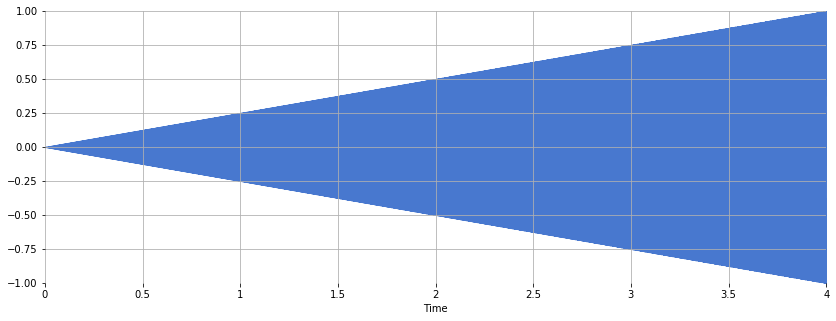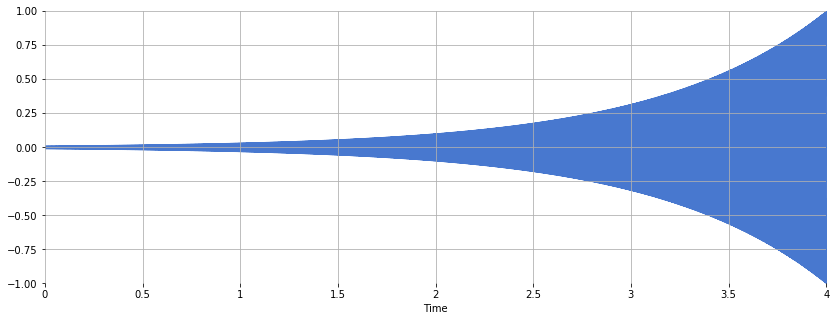# Magnitude Scaling¶

Often, the raw amplitude of a signal in the time- or frequency-domain is not as perceptually relevant to humans as the amplitude converted into other units, e.g. using a logarithmic scale.

For example, let's consider a pure tone whose amplitude grows louder linearly. Define the time variable:

In :
T = 4.0      # duration in seconds
sr = 22050   # sampling rate in Hertz
t = numpy.linspace(0, T, int(T*sr), endpoint=False)


Create a signal whose amplitude grows linearly:

In :
amplitude = numpy.linspace(0, 1, int(T*sr), endpoint=False) # time-varying amplitude
x = amplitude*numpy.sin(2*numpy.pi*440*t)


Listen:

Out:

Plot the signal:

In :
librosa.display.waveplot(x, sr=sr)

Out:
<matplotlib.collections.PolyCollection at 0x111179198>Now consider a signal whose amplitude grows exponentially, i.e. the logarithm of the amplitude is linear:

In :
amplitude = numpy.logspace(-2, 0, int(T*sr), endpoint=False, base=10.0)
x = amplitude*numpy.sin(2*numpy.pi*440*t)

Out:
In :
librosa.display.waveplot(x, sr=sr)

Out:
<matplotlib.collections.PolyCollection at 0x1111cdfd0>Even though the amplitude grows exponentially, to us, the increase in loudness seems more gradual. This phenomenon is an example of the Weber-Fechner law (Wikipedia) which states that the relationship between a stimulus and human perception is logarithmic.

## Spectrogram Visualization: Linear Amplitude¶

Let's plot a magnitude spectrogram where the colorbar is a linear function of the spectrogram values, i.e. just plot the raw values.

In :
x, sr = librosa.load('audio/latin_groove.mp3', duration=8)
ipd.Audio(x, rate=sr)

Out: